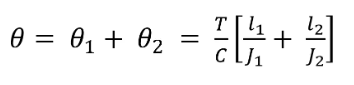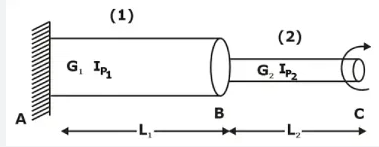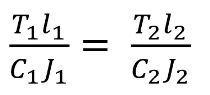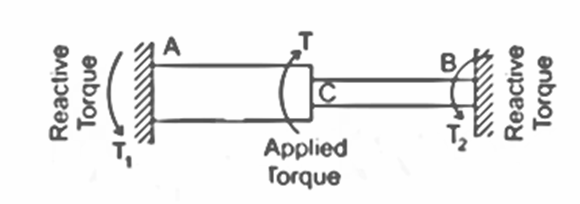Select Page

# Torsion

Contents

Torsion 1

Explain the concept of Torsion 1

Explain the assumptions in Torsion Theory 2

Describe the Torsion Equation for Circular Shafts 3

Explain Polar Moment of Inertia 3

Explain Polar Modulus of section 4

Describe and calculate Maximum Shear Stress and Torque for Solid Circular shaft 5

Describe and calculate Maximum Shear Stress and Torque for Hollow Circular shaft 6

Explain and calculate Power transmitted by Shafts 7

Define Torsional Stiffness and Torsional Rigidity 8

Explain the Significance of Strain Energy in Torsion 8

Describe the phenomena of Torsion in Series and Parallel 9

Calculate the Equivalent Bending Moment and Equivalent Torque 10

Explain the Design of Shaft 11

Calculate the Strength of the Shafts for Equal Weight and Equal Size 12

Explain the Sign Convention of Twisting Moment 13

# Explain the concept of Torsion

Torsion is a type of stress that occurs in objects when they are twisted about an axis. It is a result of the applied torque (twisting force) acting on an object, causing it to rotate around its longitudinal axis. The twisting motion creates a shear stress in the material, which can result in twisting or warping of the object if the stress exceeds the material’s strength.

Torsion is commonly seen in shafts, such as those used in machinery or drive systems. When a torque is applied to a shaft, the material on one side of the shaft is subjected to compression, while the material on the other side is subjected to tension. This creates a twisting deformation in the shaft, which is known as torsion.

The amount of torsional stress experienced by a shaft is dependent on several factors, including the magnitude of the applied torque, the length of the shaft, and the cross-sectional area of the shaft. The torsional stiffness of a shaft, which is its ability to resist twisting, is also influenced by these factors.

In order to calculate the torsional stress experienced by a shaft, engineers use equations that take into account the applied torque, the shaft’s length, cross-sectional area, and material properties. This information can be used to design shafts that can withstand the torsional loads they will be subjected to in service.

In conclusion, torsion is a type of stress that occurs in objects when they are twisted about an axis. It results from the application of a torque and creates shear stress in the material, which can cause twisting or warping of the object if the stress exceeds the material’s strength. The amount of torsional stress experienced by an object is dependent on several factors, including the magnitude of the applied torque, the length of the object, and its cross-sectional area, and can be calculated using equations based on these factors.

# Explain the assumptions in Torsion Theory

Torsion theory is a branch of mechanics that deals with the study of twisting in objects subjected to torque. There are several assumptions made in torsion theory to simplify the analysis of torsional deformation in objects. These assumptions are important to consider when calculating the torsional stress experienced by an object.

1. Pure Torsion: The first assumption made in torsion theory is that the object is subjected only to pure torsion. This means that the object experiences only twisting deformation, and no other types of deformation such as bending or axial deformation.
2. Cross-sectional Shape: The second assumption made in torsion theory is that the cross-sectional shape of the object remains constant during twisting. This means that the shape of the cross-section remains unchanged, and no warping occurs.
3. Linearity: The third assumption made in torsion theory is that the material behaviour is linear. This means that the material response is proportional to the applied load, and the material does not exhibit any nonlinear behaviour, such as yielding or plastic deformation.
4. Homogeneous Material: The fourth assumption made in torsion theory is that the object is made of a homogeneous material. This means that the material properties are the same throughout the object, and no inhomogeneities exist.
5. Thin-walled Shaft: The fifth assumption made in torsion theory is that the object is a thin-walled shaft. This means that the thickness of the wall is much smaller than the other dimensions of the object. This assumption allows for a simplified calculation of the torsional stress.

These assumptions are important to consider when analyzing torsional deformation in objects. They simplify the calculation of the torsional stress experienced by the object and make it possible to obtain accurate results in a practical manner. However, it is important to remember that these assumptions are not always valid, and the actual behaviour of an object may differ from the predictions made using torsion theory. In such cases, more advanced methods may be required to obtain accurate results.

# Describe the Torsion Equation for Circular Shafts

Torsion is the twisting of a shaft caused by an applied torque. The twisting of the shaft generates torsional stresses and strains that can lead to failure if they exceed the material’s strength. To calculate the torsional stresses and strains in a circular shaft, we use the torsion equation.

The torsion equation for circular shafts can be written as follows:

τ = T/J,

where τ is the shear stress on the cross-section of the shaft, T is the applied torque, and J is the torsional stiffness of the shaft. J is calculated using the formula:

J =

where d is the outer diameter of the shaft and is the inner diameter of the shaft if the shaft is hollow.

It is important to note that the torsion equation assumes that the cross-section of the shaft remains plane and normal to the axis of the shaft. This is known as the assumption of plane cross-sections and it is the basis of the theory of torsion. Additionally, it is also assumed that the material of the shaft is homogeneous, isotropic, and linearly elastic. These assumptions are used to simplify the calculations and make the solution more manageable. However, in reality, the assumptions may not always hold true and more complex models may be needed to accurately describe the torsional behaviour of the shaft.

# Explain Polar Moment of Inertia

The polar moment of inertia is a measure of a cross-sectional shape’s resistance to torsion. It is used to calculate the torsional stiffness, J, of a shaft and is essential in determining the torsional stresses and strains in the shaft under applied torque.

The polar moment of inertia, Ip, is defined as the sum of the products of the area of each infinitesimal element in the cross-section and the square of its distance from the axis of rotation. Mathematically, it can be expressed as:

Ip = ∫ A x r2 dA,

where A is the cross-sectional area and r is the radial distance from the axis of rotation to the element.

For a circular shaft, the polar moment of inertia can be calculated using the following formula:

Ip = (π)

where d is the outer diameter of the shaft and d0 is the inner diameter of the shaft if the shaft is hollow.

It is important to note that the polar moment of inertia depends on the shape of the cross-section and its orientation with respect to the axis of rotation. Therefore, it is crucial to accurately determine the polar moment of inertia when calculating the torsional stresses and strains in a shaft.

# Explain Polar Modulus of section

Polar Modulus of section is a property of a cross-sectional shape that is used in torsion analysis to determine the amount of torque that can be applied to a structural member without causing excessive twisting. It is calculated as the product of the polar moment of inertia (J) and the maximum shear stress (τmax) for a given cross-section. The polar modulus of the section is expressed in units of length to the fourth power (mm4, cm4, etc.).

The polar modulus of section can be used to compare the torsional stiffness of different cross-sectional shapes. A larger value of the polar modulus of section indicates that a particular cross-section is more resistant to twisting and therefore more suitable for use in applications where high torsional rigidity is required. In engineering design, the polar modulus of a section is an important factor in determining the size and shape of structural members used in torsion-critical applications, such as shafts, propeller shafts, and other rotating components.

Ip = [(π)

# Describe and calculate Maximum Shear Stress and Torque for Solid Circular shaft

Maximum shear stress and torque in a solid circular shaft are important factors in torsion analysis and determine the ability of the shaft to resist twisting. The maximum shear stress (τmax) in a circular shaft occurs at the outer surface and is proportional to the applied torque (T) and the polar moment of inertia (J) of the cross-section. The maximum shear stress can be calculated using the following equation:

τmax = T / J

The applied torque (T) is a measure of the twisting force applied to the shaft and is related to the twisting moment (M) and the shaft’s length (L) as follows:

T = M x L / 2π

The polar moment of inertia (J) is a property of the cross-sectional shape of the shaft and can be calculated using the following equation:

J = π/2 x d4 / 32 (for a solid circular shaft)

Where d is the diameter of the shaft.

It is important to note that the maximum shear stress in a circular shaft should not exceed the yield stress of the material used in the shaft to avoid failure. The torque capacity of a circular shaft can be increased by increasing its diameter or by using a material with a higher yield stress. The calculation of maximum shear stress and torque in a solid circular shaft is a crucial step in determining the size and shape of the shaft for a specific torsion-critical application.

# Describe and calculate Maximum Shear Stress and Torque for Hollow Circular shaft

The maximum shear stress and torque in a hollow circular shaft can be calculated using the following steps:

1. Determine the outside and inside diameters of the shaft (d o and d i)
2. Calculate the polar moment of inertia (J) using the formula: J =
3. Determine the shear modulus of the material (G) and the applied torque (T).
4. Calculate the maximum shear stress (τ) using the formula: τ = (T x r) / J, where r is the radial distance from the neutral axis to the outer surface of the shaft.
5. Calculate the maximum torque by multiplying the maximum shear stress by the polar moment of inertia (T = τ x J).

It is important to note that the maximum shear stress occurs at the outer surface of the shaft, while the maximum torque occurs at the neutral axis. When designing a hollow circular shaft, it is important to ensure that the maximum shear stress and torque are within the material’s allowable limits to prevent failure. Additionally, the design should also consider other factors such as the bending moment and axial load, as these can also affect the strength and stability of the shaft.

# Explain and calculate Power transmitted by Shafts

The amount of power that can be transmitted by a shaft is an important factor to consider when designing mechanical systems. Power transmission is the transfer of energy from one point to another, typically through the use of rotating shafts. The amount of power that can be transmitted by a shaft is dependent on various factors, including the diameter, length, and material properties of the shaft, as well as the torque and rotational speed.

To calculate the power transmitted by a shaft, one can use the equation:

P = T x ω

where P is the power transmitted, T is the torque applied to the shaft, and ω is the angular velocity of the shaft in radians per second.

It is important to note that the maximum power that can be transmitted by a shaft is limited by the maximum shear stress that the shaft can withstand. The maximum shear stress can be calculated using the torsion equation and the maximum torque that can be applied to the shaft.

In summary, the power transmitted by a shaft can be calculated by multiplying the torque applied to the shaft by its angular velocity. The maximum power that can be transmitted is limited by the maximum shear stress that the shaft can withstand.

# Define Torsional Stiffness and Torsional Rigidity

The terms “Torsional Stiffness” and “Torsional Rigidity” are used interchangeably to describe the resistance of a shaft to twisting. Torsional Stiffness/Rigidity measures the ability of the shaft to resist twisting due to a torque applied to its end. The higher the torsional stiffness/rigidity, the more resistance the shaft has to twisting.

The torsional stiffness of a shaft can be mathematically described by the equation: Torsional Stiffness (C) = Torque (T) / Angle of Twist (θ). Here, the torque (T) is the force applied to the shaft, and the angle of twist (θ) is the degree by which the shaft twists as a result of the torque.

In essence, torsional stiffness is a measure of the rigidity of the shaft, and is an important factor in determining the efficiency of power transmission by a shaft. Shafts with high torsional stiffness are more resistant to twisting, and thus more efficient in transmitting power. Conversely, shafts with low torsional stiffness are more prone to twisting, and thus less efficient in transmitting power.

# Explain the Significance of Strain Energy in Torsion

Strain energy is a measure of the amount of energy stored in a material due to the application of an external load. In torsion, the external load is a twisting force, which causes the material to deform. The amount of energy stored in the material due to this deformation is referred to as the strain energy.

Strain energy is important in torsion because it provides a way to quantify the energy that is being stored in a shaft as it is subjected to a twisting force. This information can be used to design shafts that can withstand the loads they will be subjected to in service, as well as to calculate the power that can be transmitted by a shaft.

The strain energy in torsion can be calculated using the equation U = (T2 x J) / (2 x G), where T is the torque applied to the shaft, J is the polar moment of inertia of the cross-section, and G is the shear modulus of the material. This equation can be used to determine the maximum strain energy that can be stored in a shaft, which in turn can be used to calculate the maximum torque that the shaft can transmit.

In summary, the strain energy in torsion is important because it provides a way to quantify the amount of energy that is being stored in a shaft as it is subjected to a twisting force. This information is essential for designing shafts that can withstand the loads they will be subjected to in service and for calculating the power that can be transmitted by a shaft.

# Describe the phenomena of Torsion in Series and Parallel

Torsion in series and parallel refers to the behaviour of two or more shafts that are subjected to torsion and are connected to form a system. In such a system, the shafts may either be in series or in parallel, depending on the way they are connected.

When shafts are connected in series, the total torque is distributed equally among the shafts. The total torsional stiffness of the system is equal to the sum of the torsional stiffnesses of individual shafts. The maximum shear stress in each shaft is proportional to the total torque applied to the system and the polar moment of inertia of each shaft.On the other hand, when shafts are connected in parallel, the total torque is distributed proportionally among the shafts according to their torsional stiffnesses. The total torsional stiffness of the system is equal to the reciprocal of the sum of the reciprocals of the torsional stiffnesses of individual shafts. The maximum shear stress in each shaft is proportional to the applied torque and the polar moment of inertia of each shaft.

It is important to understand the concept of torsion in series and parallel as it is relevant in designing mechanical systems, especially when multiple shafts are involved. The choice between series and parallel connection of shafts depends on the design requirements, such as the amount of torque to be transmitted, the torsional stiffness desired, and the maximum shear stress that each shaft can withstand.# Calculate the Equivalent Bending Moment and Equivalent Torque

The equivalent bending moment and equivalent torque are important parameters when considering torsion in series and parallel. These calculations are necessary to determine the overall effect of torsion on a structure.

The equivalent bending moment is the total bending moment that would produce the same strain energy as the torsion in a structure. It is determined by multiplying the torsion torque by the distance from the neutral axis to the outer surface of the structure. This calculation provides an estimate of the total bending moment that the structure is subjected to due to torsion.

The equivalent torque is the total torque that would produce the same torsional strain energy as the combined torsion in a structure. This calculation involves finding the total torsional strain energy and then dividing it by the total length of the structure.

To determine the equivalent bending moment and equivalent torque, it is necessary to have a clear understanding of the geometry of the structure and the forces acting on it. The calculations involve using equations from torsion theory, such as the torsion equation for circular shafts and the equation for maximum shear stress and torque for both solid and hollow circular shafts.
Te=

Overall, the equivalent bending moment and equivalent torque are crucial parameters for engineers and designers when considering torsion in structures. These calculations provide a more accurate estimate of the overall effect of torsion on a structure and can help to prevent failure due to excessive bending or twisting.

# Explain the Design of Shaft

The design of a shaft involves ensuring that it can withstand the loads and stresses that will be applied to it during its intended use. To design a shaft, one needs to consider various factors such as the size and shape of the shaft, the type and amount of loading it will experience, and the materials used to make the shaft. The following are the steps involved in the design of a shaft:

1. Determining the loads and stresses: The first step in the design of a shaft is to determine the loads and stresses that it will experience. This information can be obtained from engineering calculations, simulations, or actual testing.
2. Selecting the material: The next step is to select the material that will be used to make the shaft. The material selected should have the necessary strength and stiffness to withstand the loads and stresses that will be applied to the shaft.
3. Selecting the size and shape: Based on the loads and stresses, the size and shape of the shaft can be selected. The size and shape of the shaft are important because they determine the stiffness and strength of the shaft.
4. Calculating the strength: Once the size and shape have been determined, the strength of the shaft can be calculated. This calculation involves determining the maximum stress that the shaft will experience, and then comparing it to the allowable stress for the material selected.
5. Design verification: The final step is to verify the design of the shaft. This involves performing simulations, testing, or other analysis to ensure that the shaft will be able to withstand the loads and stresses that it will experience in its intended use.

It is important to note that the design of a shaft is a complex process that requires a thorough understanding of the loads and stresses that it will experience, as well as the materials and construction methods used to make the shaft. A properly designed shaft is essential to ensure the safety and reliability of mechanical systems and structures.

# Calculate the Strength of the Shafts for Equal Weight and Equal Size

The calculation of the strength of shafts is an important aspect of mechanical engineering as it determines the ability of the shaft to withstand loads and deformations.

To calculate the strength of shafts for equal weight and equal size, one must consider the following factors:

1. Material Properties: The strength of the shaft is determined by the properties of the material from which it is made. For example, materials like steel have a higher strength compared to materials like aluminium.
2. Cross-sectional Area: The strength of a shaft is directly proportional to its cross-sectional area. Hence, a larger cross-sectional area results in a stronger shaft.
3. Diameter: The diameter of the shaft is also an important factor in determining its strength. A larger diameter results in a stronger shaft as the cross-sectional area increases with the diameter.
4. Length: The length of the shaft is also an important factor in determining its strength. A longer shaft is more likely to bend or deform compared to a shorter shaft. Hence, longer shafts require a higher strength to withstand the same load compared to shorter shafts.
5. Load: The strength of the shaft is also dependent on the load it is expected to carry. A shaft must be strong enough to carry the maximum expected load without breaking or deforming excessively.

Once the above factors have been considered, the strength of the shafts for equal weight and equal size can be calculated using the following formula:

Strength = (π x D2 x T x S) / 4

Where:

π is the mathematical constant pi

D is the diameter of the shaft

T is the thickness of the shaft

S is the ultimate tensile strength of the material

It is important to note that the above formula is only a rough estimate of the strength of the shaft. In actual engineering practice, more complex formulas and calculations are used to determine the strength of a shaft.

# Explain the Sign Convention of Twisting Moment

Twisting moment, also known as torsional moment or torque, is a measure of the rotational force applied to a shaft. Understanding the sign convention of the twisting moment is important for accurately analysing the behaviour of shafts subjected to torsional loads.

The sign convention of the twisting moment follows the right-hand rule. According to this rule, if the thumb of the right hand is pointed in the direction of the shaft axis, the fingers of the hand curl in the direction of the positive twisting moment. The negative twisting moment is then in the opposite direction.

The sign convention can be used to determine the direction of the twisting moment in a shaft. For example, if a shaft is subjected to a clockwise twisting moment, it is considered positive. On the other hand, if the shaft is subjected to a counterclockwise twisting moment, it is considered negative.

It is important to note that the sign convention of the twisting moment is only used for analysis purposes and does not have a physical significance. The magnitude of the twisting moment, regardless of its sign, indicates the amount of rotational force applied to the shaft and the potential for deformation or failure.

In conclusion, understanding the sign convention of the twisting moment is an important aspect of mechanical engineering as it allows for accurate analysis of the behaviour of shafts subjected to torsional loads.